## 23.11.14

### Function : Excercise-12 : Sum of all even elements from an Array

Write a program of function to Sum of all even elements from an Array. The initial value of the array must take from main ( ) as input

#### 22 comments:

1.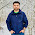#include

#include
using namespace std;
int main()
{
int a ;
int i , s;

s=0;

cout<<"enter values in array:\n" ;
for(i=0 ; i<10 ; i++)
{
cin>>a[i] ;

if(a[i]%2==0)
{
s = s + a[i] ;
}
}

cout<<"Sum of all even numbers in the array is:"<<s ;
getch();
}

1.your answer is not relevant with question format. I asked you to solve using function(user defined function). But this is a correct program. Submit again using function.

2.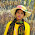#include
using namespace std;
int arraysum(int a[],int len);
main()
{
int len,j;
cout<<"the array size is:"<>len;
int a[len];
cout<<"\nyour values:";
for(j=0;j>a[j];

arraysum(a,len);
}
int arraysum(int b[],int n)
{
int i,sum=0;
for(i=0;i<n;i++)
{
if(b[i]%2==0)
{
cout<<"Even: "<<b[i]<<endl;
sum+=b[i];
}
}
cout<<sum;
}

1.its correct.

3.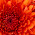my programme published half,what is the problem sir??plz.

1.Don't worry. I think your internet line is slow. Try after sometimes.

4.Dear Sir, Please visit:
http://itlearn24.blogspot.com/2014/11/sum-of-all-even-elements-from-array.html

1.5.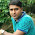name-Habibur Rahman
id-201421091
batch-46
#include
#include
using namespace std;
int main()
{
int G ;
int i , m;

m=0;

cout<<"enter array:\n" ;
for(i=0 ; i<10 ; i++)
{
cin>>G[i] ;

if(G[i]%2==0)
{
m = m + G[i] ;
}
}

cout<<"Sum of all even numbers is:"<<m ;
getch();
}

1.you didn't used function. try to follow question. advised to submit again.

6.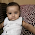#include
using namespace std;

void getsum(int digit)
{
int a,sum=0;
cout<<"Enter "<>a[i];
}
for (int i=0;i>digit;
getsum(digit);
}

7.Dear Sir, I have upload full program but not show.

8.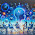This comment has been removed by the author.

9.Dear Sir, I have upload full program but not some show this program

10.Mohammad Ashraful Hasan sobuj
CSE-46(Dip)
ID=201420656

#include
using namespace std;

class evensum
{
public:
void setvalue(int v)
{
value =v;
}
protected: int value;

};

class mysum: public evensum
{
public:
void getsum()
{
int temperature,i,a,sum=0;
temperature=value;
cout<<"Enter "<>a[i];
}
for (i=0;i>v;
xy.setvalue(v);
xy.getsum();
return 0;
}

11.Dear Sir, I have upload full program but not show some this program

12.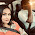This comment has been removed by the author.

13.#include
using namespace std;
class pa
{
int i,sum=0;
public:
int an(int a[],int n)
{
for(i=0;i<n;i++)
{
if (a[i]%2==0)
sum+=a[i];
}
return sum;
}
};

14.main()
{
pa pb;
int i,n;
cout<<"Enter length"<>n;
int a[n];
cout<<"Enter the values"<>a[i];
cout<<"The sum is ";
cout<<pb.an(a,n);
}

15.due to publishing problem i couldnt posted my full program code here,so i divided it into two parts...and posted 1st half 1st,2nd half below

16.#include
#include
void main()
{
int num;
int i , esum , osum , N;

clrscr();
esum = 0;
osum = 0;
printf("Enter the size of Array\n");
scanf("%d" , &N);

printf(" Enter Array elements\n");
for(i=0 ; i<N ; i++)
{
scanf("%d" , &num[i]);
}

for(i=0 ; i<N ; i++)
{
if((num[i] %2) ==0)
{
esum+ = num[i];
}
else
{
osum+ = num[i];
}
}

17.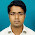md.Abul kashem
roll-201410856

#include
using namespace std;
int arraysum(int r[],int len);
main()
{
int len,j;
cout<<"the array size is:"<>len;
int r[len];
cout<<"\nyour values:";
for(j=0;j>r[j];

arraysum(r,len);
}
int arraysum(int b[],int n)
{
int i,sum=0;
for(i=0;i<n;i++)
{
if(b[i]%2==0)
{
cout<<"Even: "<<b[i]<<endl;
sum+=b[i];
}
}
cout<<sum;
}

Comment Here